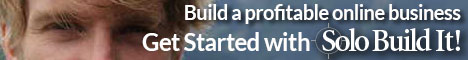# Basic Math Words Beginning with L

Least Common Denominator (LCD): The smallest denominator possible for two or more fractions which we want to write in equivalent forms, all with the same denominator.

Least common multiple (LCM): The smallest number that can be exactly divided by all the given numbers.

Less than (<): It is given by this symbol “<”, and denotes one quantity is smaller than another.

Less than or equal to:  Represented by this symbol “≤”, and denotes one quantity is equal to or smaller to another.

Like Terms: Terms that have exactly the same variables raised to exactly the same powers.

Line: A line is the path formed by a moving point.

Line Graph:  A graph that connects the points that represent the quantities in two different scales with a line.

Linear Equation: An equation whose graph forms a straight line.

Lowest Terms (Simplest Form):  When a fraction is written with the smallest possible numbers for both its numerator and denominator.